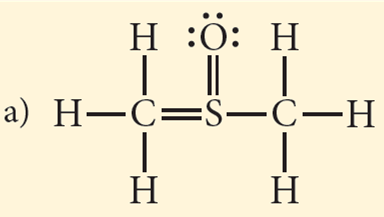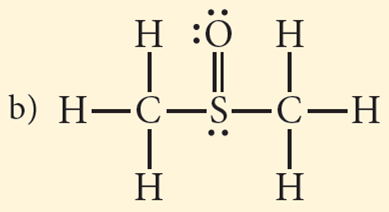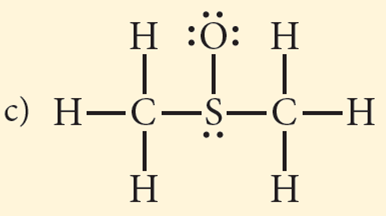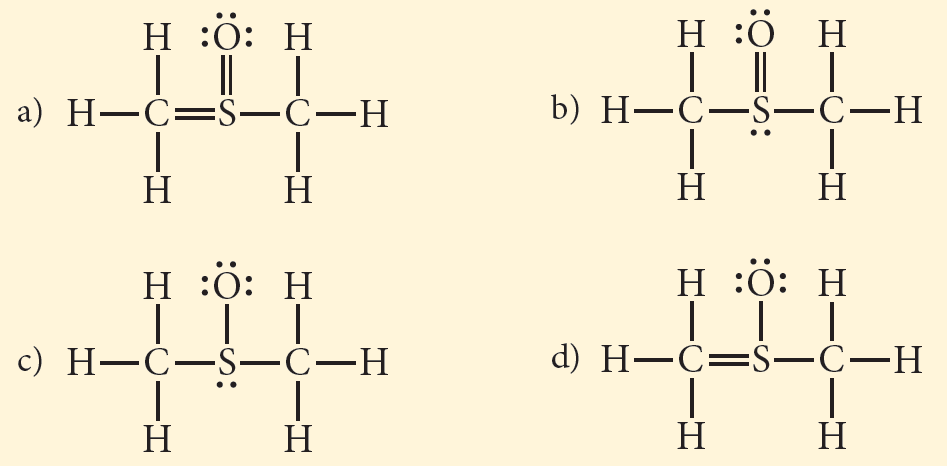# Problem: Use formal charge to choose the best Lewis structure for CH3SOCH3.

###### FREE Expert Solution

Recall that the formula for the formal charge is:Calculating for the formal charge of each atom:

F.C. of H = 1 – (1 + 0) = 0

F.C. of C = 4 – (4 + 0) = 0

F.C. of S = 6 – (5 + 0) = +1

F.C. of O = 6 – (2 + 6) = –2Calculating for the formal charge of each atom:

F.C. of H = 1 – (1 + 0) = 0

F.C. of C = 4 – (4 + 0) = 0

F.C. of S = 6 – (4 + 2) = 0

F.C. of O = 6 – (2 + 4) = 083% (216 ratings)###### Problem Details

Use formal charge to choose the best Lewis structure for CH3SOCH3.Frequently Asked Questions

What scientific concept do you need to know in order to solve this problem?

Our tutors have indicated that to solve this problem you will need to apply the Formal Charge concept. You can view video lessons to learn Formal Charge. Or if you need more Formal Charge practice, you can also practice Formal Charge practice problems.

What professor is this problem relevant for?

Based on our data, we think this problem is relevant for Professor Singh's class at KSU.